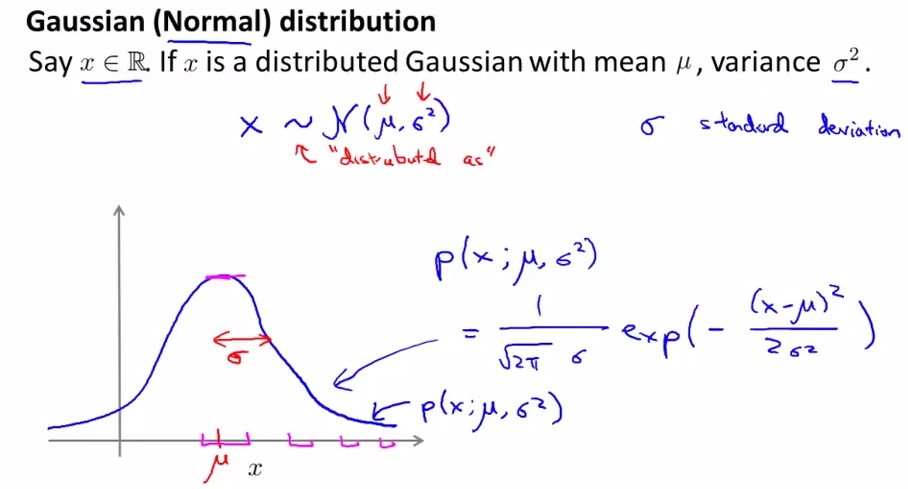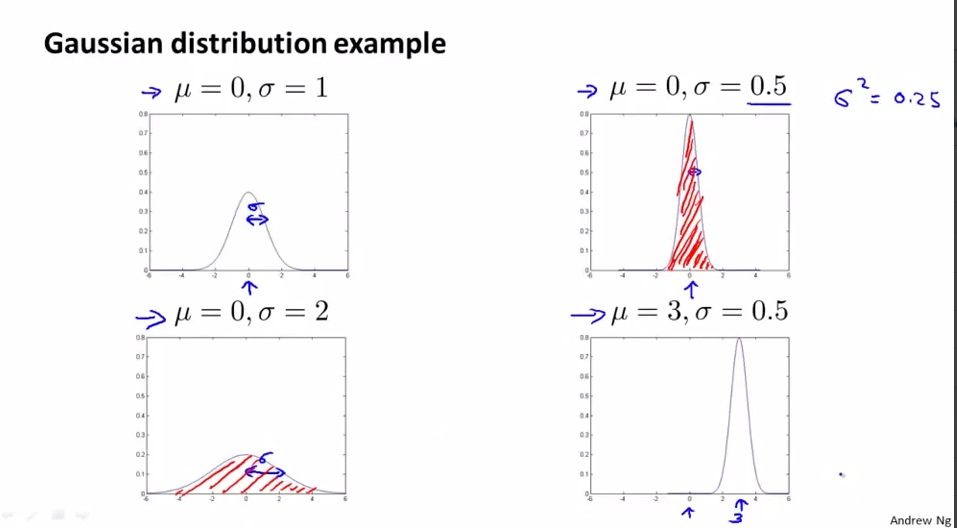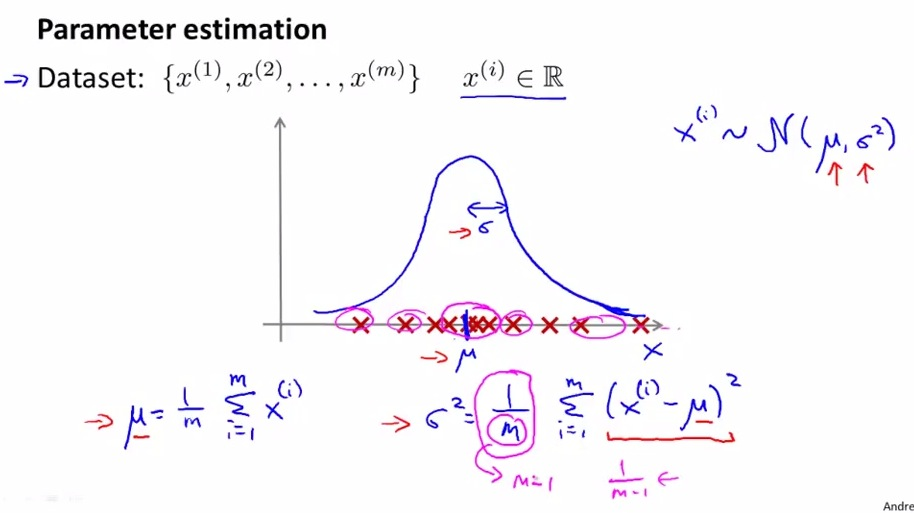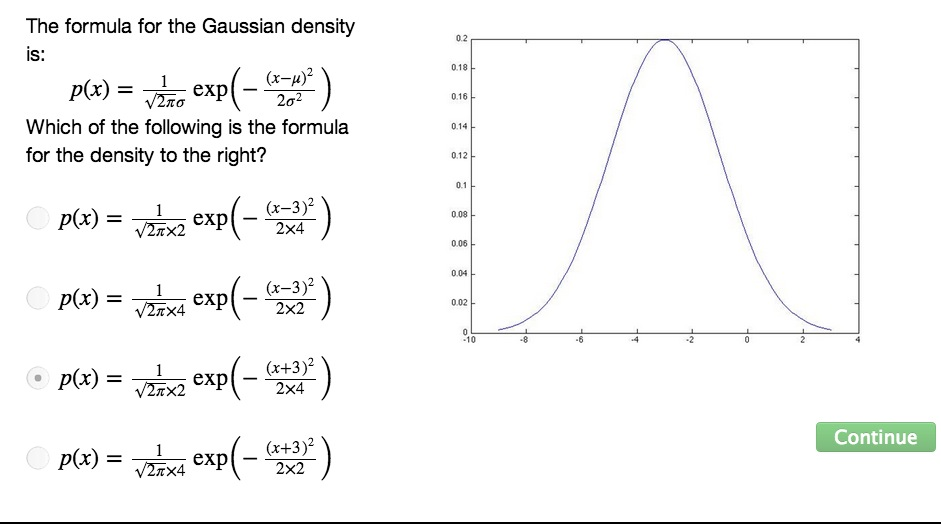• Gaussian/Normal Distribution• sigma = one standard deviation
• Gaussian = Range of Doubtness
• probability x is depend on the mew and sigma parameters
• Fixed formula and no need to be remembered• Less the sigma, higher the probability, make a narrower and higher graph
• more sigma, more doubtness, fatter gaussian distribution• we want to plot the Gaussian distribution of every examples
• The problem is we don't know what to set mew and sigma squared parameter
• Or maybe we can set the parameters?
• We can set the parameters based on the distance of the parameters gathered
• mew = average of the example
• sigma squared = is the range of error. how much the variance of x from the average(mew) value. Summation of range error between x and amew
• Maximum Likelihood Estimate is the name of formula above
• ML tend to use m training set rather than m-1SUMMARY

• Given a training set, now we can set mew and sigma for Gaussian Distribution
• Next how to implement GD in Anomaly Detection